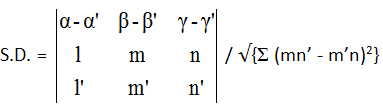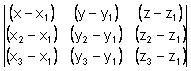# Find the Value of Lambda.For the following given data.Plz answer my question asa fast as u canSaurabh Koranglekar
3 years ago
Dear student

Regards
3 years ago• General equation of a plane is ax + by + cz + d = 0.

• Equation of the plane in Normal form is lx + my + nz = p where p is the length of the normal from the origin to the plane and (l, m, n) be the direction cosines of the normal.

• The equation of the plane passing through P(x1, y1, z1) and having direction ratios (a, b, c) for its normal is a (x – x1) + b (y – y1) + c (z – z1) = 0

• The equation of the plane passing through three non-collinear points (x1, y1, z1), (x2, y2, z2) and (x3, y3 , z3) is

•